## Paired t-Test

Given two paired setsand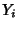ofmeasured values, the paired-test determines if they differ from each other in a significant way. Let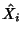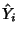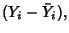then defineby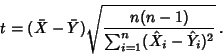This statistic has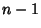Degrees of Freedom.

A table of Student's t-Distribution confidence interval can be used to determine the significance level at which two distributions differ.

See also Fisher Sign Test, Hypothesis Testing, Student's t-Distribution, Wilcoxon Signed Rank Test

References

Goulden, C. H. Methods of Statistical Analysis, 2nd ed. New York: Wiley, pp. 50-55, 1956.